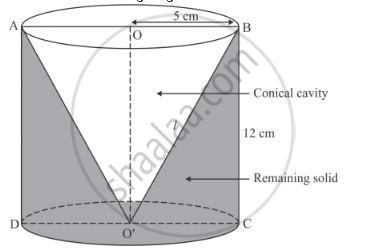Share

# A Conical Hole is Drilled in a Circular Cylinder of Height 12 Cm and Base Radius 5 Cm. the Height and the Base Radius of the Cone Are Also the Same. Find the Whole Surface and Volume of the Remaining Cylinder. - CBSE Class 10 - Mathematics

ConceptSurface Areas and Volumes Examples and Solutions

#### Question

A conical hole is drilled in a circular cylinder of height 12 cm and base radius 5 cm. The height and the base radius of the cone are also the same. Find the whole surface and volume of the remaining cylinder.

#### Solution

Given that:

r = 5 cm

h = 12 cm

We have the following diagramSlant height of cone is given by

l=sqrt(r^2+h^2)

=sqrt(5^2+12^2

= 13 cm

The total surface area of the remaining part is given by

S=2pirh+pir^2+pirl

2xxpixx5xx12+pixx5^2+pixx5xx13

= 120π+25π+65π

=210π cm2

The volume of the remaining part is given by

V=pir^2h-1/3pir^2h

=2/3pir^2h

=2/3xxpixx5^2xx12

= 200π cm3

Hence, S =210π cm2. V = 200π cm3

Is there an error in this question or solution?

#### APPEARS IN

RD Sharma Solution for 10 Mathematics (2018 to Current)
Chapter 14: Surface Areas and Volumes
Ex. 14.20 | Q: 10 | Page no. 61

#### Video TutorialsVIEW ALL 

Solution A Conical Hole is Drilled in a Circular Cylinder of Height 12 Cm and Base Radius 5 Cm. the Height and the Base Radius of the Cone Are Also the Same. Find the Whole Surface and Volume of the Remaining Cylinder. Concept: Surface Areas and Volumes Examples and Solutions.
S